Anzeige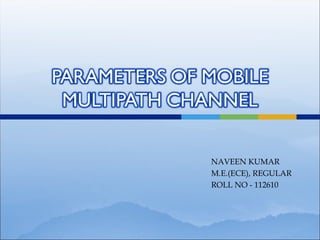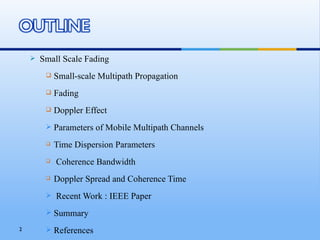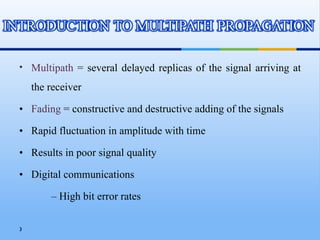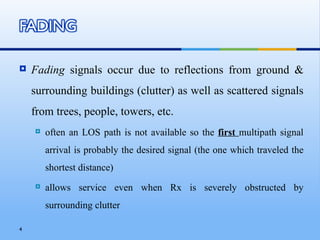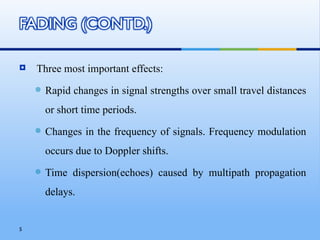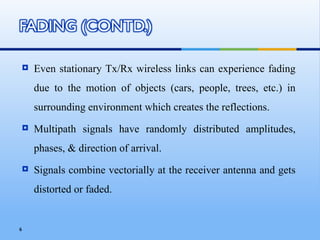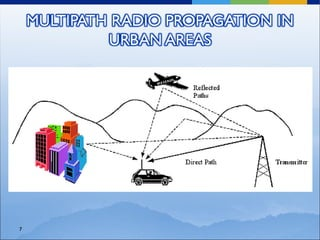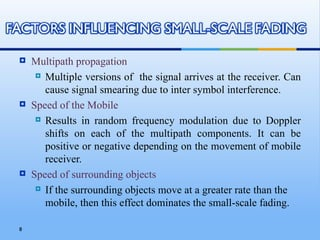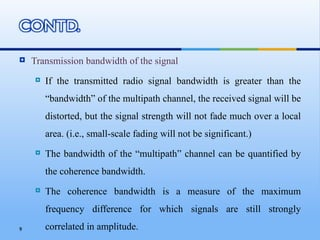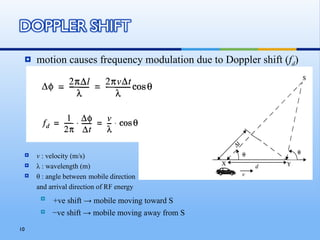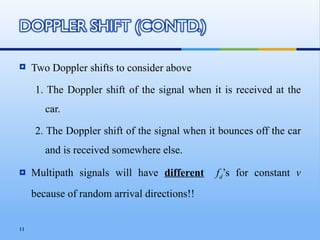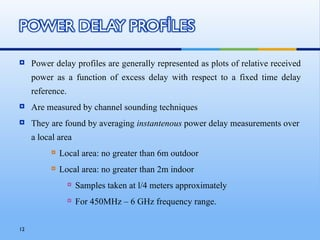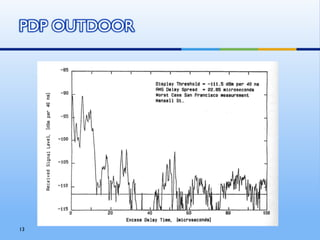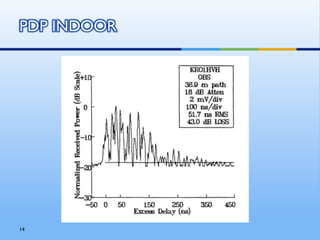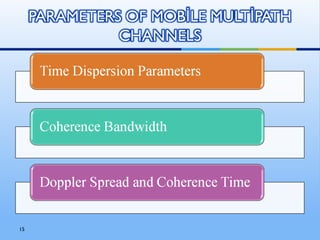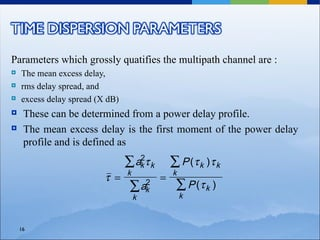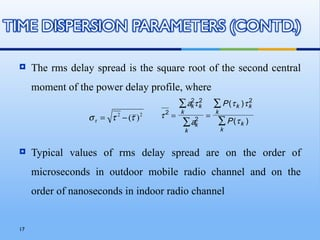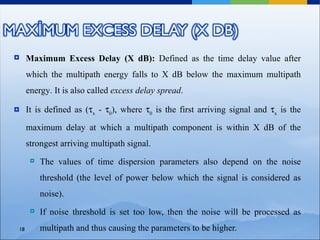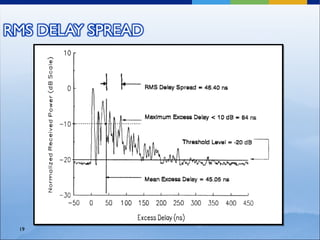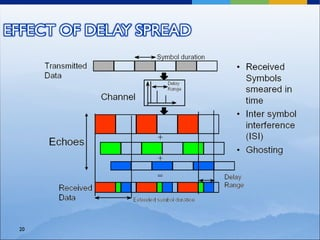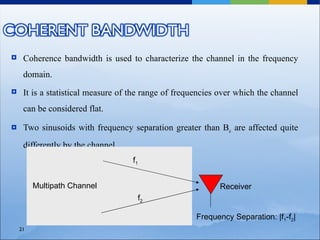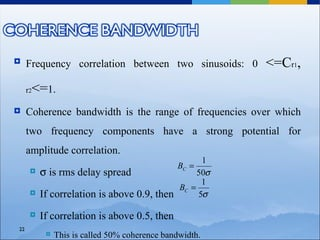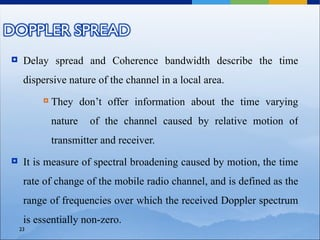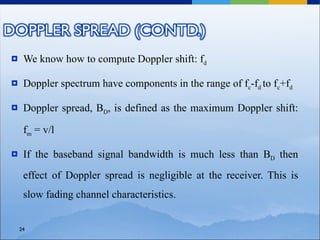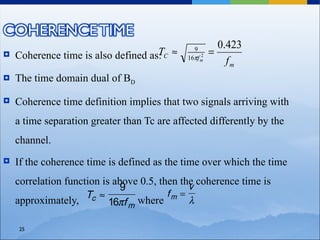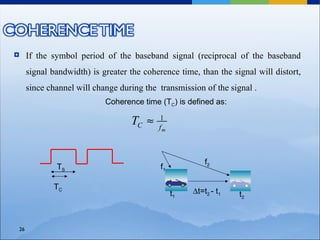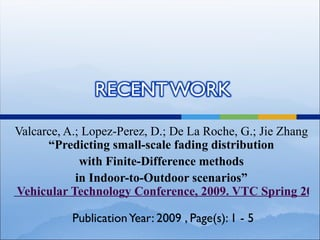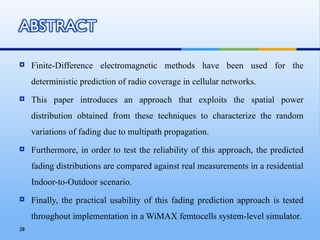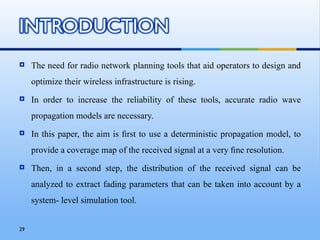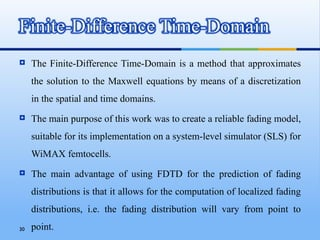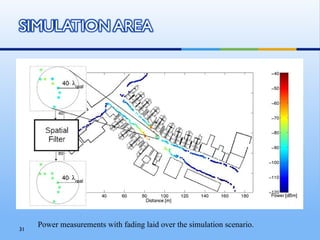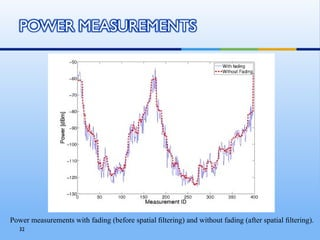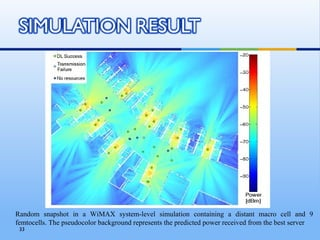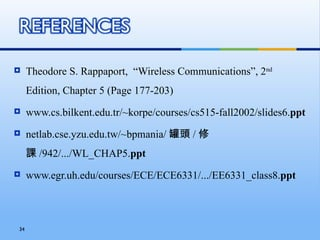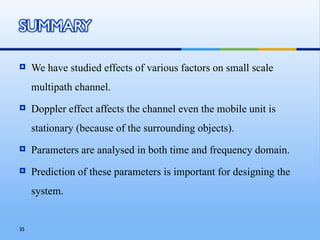1 von 36
Anzeige

### Parameters of multipath channel

1. NAVEEN KUMAR M.E.(ECE), REGULAR ROLL NO - 112610
2. Small Scale Fading  Small-scale Multipath Propagation  Fading  Doppler Effect  Parameters of Mobile Multipath Channels  Time Dispersion Parameters  Coherence Bandwidth  Doppler Spread and Coherence Time  Recent Work : IEEE Paper  Summary 2  References
3. • Multipath = several delayed replicas of the signal arriving at the receiver • Fading = constructive and destructive adding of the signals • Rapid fluctuation in amplitude with time • Results in poor signal quality • Digital communications – High bit error rates 3
4. Fading signals occur due to reflections from ground & surrounding buildings (clutter) as well as scattered signals from trees, people, towers, etc.  often an LOS path is not available so the first multipath signal arrival is probably the desired signal (the one which traveled the shortest distance)  allows service even when Rx is severely obstructed by surrounding clutter 4
5. Three most important effects:  Rapid changes in signal strengths over small travel distances or short time periods.  Changes in the frequency of signals. Frequency modulation occurs due to Doppler shifts.  Time dispersion(echoes) caused by multipath propagation delays. 5
6. Even stationary Tx/Rx wireless links can experience fading due to the motion of objects (cars, people, trees, etc.) in surrounding environment which creates the reflections.  Multipath signals have randomly distributed amplitudes, phases, & direction of arrival.  Signals combine vectorially at the receiver antenna and gets distorted or faded. 6
7. 7
8. Multipath propagation  Multiple versions of the signal arrives at the receiver. Can cause signal smearing due to inter symbol interference.  Speed of the Mobile  Results in random frequency modulation due to Doppler shifts on each of the multipath components. It can be positive or negative depending on the movement of mobile receiver.  Speed of surrounding objects  If the surrounding objects move at a greater rate than the mobile, then this effect dominates the small-scale fading. 8
9. Transmission bandwidth of the signal  If the transmitted radio signal bandwidth is greater than the “bandwidth” of the multipath channel, the received signal will be distorted, but the signal strength will not fade much over a local area. (i.e., small-scale fading will not be significant.)  The bandwidth of the “multipath” channel can be quantified by the coherence bandwidth.  The coherence bandwidth is a measure of the maximum frequency difference for which signals are still strongly 9 correlated in amplitude.
10. motion causes frequency modulation due to Doppler shift (fd)  v : velocity (m/s)  λ : wavelength (m)  θ : angle between mobile direction and arrival direction of RF energy  +ve shift → mobile moving toward S  −ve shift → mobile moving away from S 10
11. Two Doppler shifts to consider above 1. The Doppler shift of the signal when it is received at the car. 2. The Doppler shift of the signal when it bounces off the car and is received somewhere else.  Multipath signals will have different fd’s for constant v because of random arrival directions!! 11
12. Power delay profiles are generally represented as plots of relative received power as a function of excess delay with respect to a fixed time delay reference.  Are measured by channel sounding techniques  They are found by averaging instantenous power delay measurements over a local area  Local area: no greater than 6m outdoor  Local area: no greater than 2m indoor  Samples taken at l/4 meters approximately  For 450MHz – 6 GHz frequency range. 12
13. 13
14. 14
15. 15
16. Parameters which grossly quatifies the multipath channel are :  The mean excess delay,  rms delay spread, and  excess delay spread (X dB)  These can be determined from a power delay profile.  The mean excess delay is the first moment of the power delay profile and is defined as ∑ ak τ k 2 ∑ P (τ k )τ k k k τ = = ∑ ak 2 ∑ P (τ k ) k k 16
17. The rms delay spread is the square root of the second central moment of the power delay profile, where ∑ ak τ k 2 2 ∑ P (τ k )τ k 2 k k σ τ = τ 2 − (τ ) 2 τ2 = = ∑ ak 2 ∑ P (τ k ) k k  Typical values of rms delay spread are on the order of microseconds in outdoor mobile radio channel and on the order of nanoseconds in indoor radio channel 17
18. Maximum Excess Delay (X dB): Defined as the time delay value after which the multipath energy falls to X dB below the maximum multipath energy. It is also called excess delay spread.  It is defined as (τx - τ0), where τ0 is the first arriving signal and τx is the maximum delay at which a multipath component is within X dB of the strongest arriving multipath signal.  The values of time dispersion parameters also depend on the noise threshold (the level of power below which the signal is considered as noise).  If noise threshold is set too low, then the noise will be processed as 18 multipath and thus causing the parameters to be higher.
19. 19
20. 20
21. Coherence bandwidth is used to characterize the channel in the frequency domain.  It is a statistical measure of the range of frequencies over which the channel can be considered flat.  Two sinusoids with frequency separation greater than Bc are affected quite differently by the channel. f1 Multipath Channel Receiver f2 Frequency Separation: |f1-f2| 21
22. Frequency correlation between two sinusoids: 0 <=Cr1, r2 <=1.  Coherence bandwidth is the range of frequencies over which two frequency components have a strong potential for amplitude correlation. 1 BC =  σ is rms delay spread 50σ 1 BC =  If correlation is above 0.9, then 5σ  If correlation is above 0.5, then 22  This is called 50% coherence bandwidth.
23. Delay spread and Coherence bandwidth describe the time dispersive nature of the channel in a local area.  They don’t offer information about the time varying nature of the channel caused by relative motion of transmitter and receiver.  It is measure of spectral broadening caused by motion, the time rate of change of the mobile radio channel, and is defined as the range of frequencies over which the received Doppler spectrum is essentially non-zero. 23
24. We know how to compute Doppler shift: fd  Doppler spectrum have components in the range of fc-fd to fc+fd  Doppler spread, BD, is defined as the maximum Doppler shift: fm = v/l  If the baseband signal bandwidth is much less than BD then effect of Doppler spread is negligible at the receiver. This is slow fading channel characteristics. 24
25. 0.423 Coherence time is also defined as: C ≈ T = 9  2 16πf m fm  The time domain dual of BD  Coherence time definition implies that two signals arriving with a time separation greater than Tc are affected differently by the channel.  If the coherence time is defined as the time over which the time correlation function is above 0.5, then the coherence time is v 9 Tc ≈ fm = approximately, 16πf m where λ 25
26. If the symbol period of the baseband signal (reciprocal of the baseband signal bandwidth) is greater the coherence time, than the signal will distort, since channel will change during the transmission of the signal . Coherence time (TC) is defined as: TC ≈ 1 fm f2 TS f1 TC t1 ∆t=t2 - t1 t2 26
27. Valcarce, A.; Lopez-Perez, D.; De La Roche, G.; Jie Zhang “Predicting small-scale fading distribution with Finite-Difference methods in Indoor-to-Outdoor scenarios” Vehicular Technology Conference, 2009. VTC Spring 2009 Publication Year: 2009 , Page(s): 1 - 5
28. Finite-Difference electromagnetic methods have been used for the deterministic prediction of radio coverage in cellular networks.  This paper introduces an approach that exploits the spatial power distribution obtained from these techniques to characterize the random variations of fading due to multipath propagation.  Furthermore, in order to test the reliability of this approach, the predicted fading distributions are compared against real measurements in a residential Indoor-to-Outdoor scenario.  Finally, the practical usability of this fading prediction approach is tested throughout implementation in a WiMAX femtocells system-level simulator. 28
29. The need for radio network planning tools that aid operators to design and optimize their wireless infrastructure is rising.  In order to increase the reliability of these tools, accurate radio wave propagation models are necessary.  In this paper, the aim is ﬁrst to use a deterministic propagation model, to provide a coverage map of the received signal at a very ﬁne resolution.  Then, in a second step, the distribution of the received signal can be analyzed to extract fading parameters that can be taken into account by a system- level simulation tool. 29
30. The Finite-Difference Time-Domain is a method that approximates the solution to the Maxwell equations by means of a discretization in the spatial and time domains.  The main purpose of this work was to create a reliable fading model, suitable for its implementation on a system-level simulator (SLS) for WiMAX femtocells.  The main advantage of using FDTD for the prediction of fading distributions is that it allows for the computation of localized fading distributions, i.e. the fading distribution will vary from point to 30 point.
31. 31 Power measurements with fading laid over the simulation scenario.
32. Power measurements with fading (before spatial ﬁltering) and without fading (after spatial ﬁltering). 32
33. Random snapshot in a WiMAX system-level simulation containing a distant macro cell and 9 femtocells. The pseudocolor background represents the predicted power received from the best server 33
34. Theodore S. Rappaport, “Wireless Communications”, 2nd Edition, Chapter 5 (Page 177-203)  www.cs.bilkent.edu.tr/~korpe/courses/cs515-fall2002/slides6.ppt  netlab.cse.yzu.edu.tw/~bpmania/ 罐頭 / 修 課 /942/.../WL_CHAP5.ppt  www.egr.uh.edu/courses/ECE/ECE6331/.../EE6331_class8.ppt 34
35. We have studied effects of various factors on small scale multipath channel.  Doppler effect affects the channel even the mobile unit is stationary (because of the surrounding objects).  Parameters are analysed in both time and frequency domain.  Prediction of these parameters is important for designing the system. 35
36. 36